#### Bound States, Physics tutorial

Introduction to Bound States:

You would remind that a body in the simple harmonic motion bounces backward and forward between the two points where the total or net mechanical energy, 'E', of the body is equivalent to the potential energy. As the kinetic energy becomes zero, the body should turn back. In just similar manner, a quantum-mechanical oscillator is a particle within an infinite potential well. You must observe that certainly, the solutions will be sinusoidal, just the manner it is by a harmonic oscillator. Such a state is an illustration of a bound state. More particularly, we shall state a system is in the bound state if, E < V (-∞). As such the wave function comprised should die at infinity, that is, Ψ(x) → 0 as x → ± ∞. E > V (- ∞) or/and E > V (+ ∞) is termed as a scattering state. The other manner of seeing a bound state is to state that the particle is subjected to the attracting potential.

For a bound state, the given conditions apply:

a) Ψ is continuous across the boundary

b) The first derivative, Ψ', is continuous across the boundary

Particle in an infinite potential well:

This is as well termed as a particle in a box. The figure shown below describes a particle in an infinite potential well.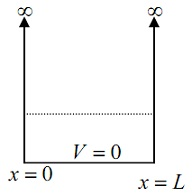The above figure is the infinite square well potential confining a particle to a region having width 'L'.

Within the well, the potential is zero whereas outside the well, the potential is infinite. We anticipate that the wave function outside the well will be equivalent to zero.

We remember the Schrodinger equation: (∂2Ψ/∂x2) + (8π2m/h2) (E - V) Ψ = 0

If, V = 0,

(d2Ψ/dx2) + (8π2m/h2) EΨ = 0

It can be written as:

(d2Ψ/dx2) + k2Ψ = 0

The common solution of this equation is:

Ψ(x) = c1eikx + c2e-ikx

Here c1 and c2 are the constants to be determined, subject to the boundary conditions and i = √-1.

As,

a) Ψ(x) = 0 for x = 0

Ψ(0) = c1eik0 + c2e-ik0 = 0

=> c1 + c2 = 0 => c1 = -c2 = c

=> Ψ(x) = c (eikx - e-ikx) = A sin kx

Here A = 2ic

b) Ψ(x) = 0 for x = L

A sin kL = 0 => kL = nπ, where n = 0, 1, 2,........

It obeys that the values of k are quantized, in such a way that kn = nπ/L

Therefore, the corresponding energy En = (n2h2)/(8mL2), and the wave-function corresponding to this energy is Ψn(x) = An sin (nπx/L).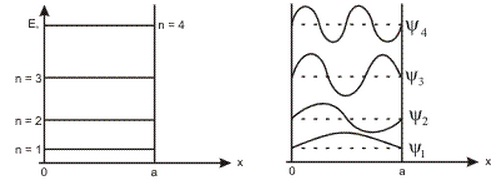An, termed as the normalization constant is obtained through applying the normalization condition:

-∞ |Ψ(x)|2 dx = 1

That is, An2 oL sin2 (nπx/L) dx = 1

Or An2 x 1/2 = 1,

From which it follows that,

An = √ (2/L)

We can thus write:

Ψn(x) = √ (2/L) sin (nπx/L) for 0 ≤ x ≤ L; n = 1, 2, 3....

The Finite Potential Well: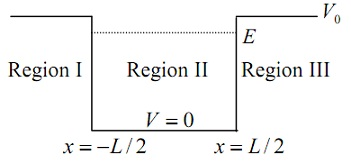In case of a finite potential well, the wave-functions 'spill over' to the area outside the potential well.

Region 1:

x ≤ - L/2, V(x) = Vo, Putting into time independent Schrodinger equation: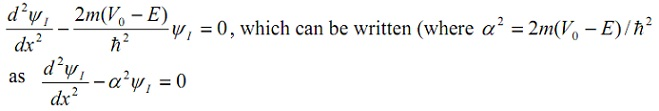The above can be written in the form:

ΨI = Ceαx + De-αx

Though, we can't let an exponentially growing term; so we set D equivalent to zero.

ΨI = Ceαx

Region 2:

- (L/2) ≤ x ≤ (L/2), V(x) = 0, putting it into the time independent Schrodinger equation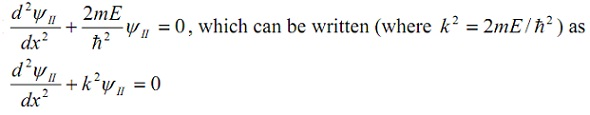The above can be written in the form:

ΨII = A sin kx + B cos kx

This comprises of an odd and an even solution.

We at first consider the even solution: ΨII = B cos kx

Region 3:

x ≥ L/2, V(x) = Vo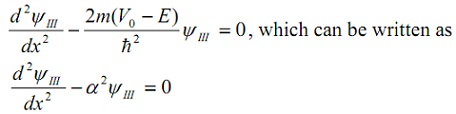The above can be written in the form:

ΨIII = Feαx + Ge-αx

Though, we can't let an exponentially growing term; therefore we set F equivalent to zero.

ΨIII = Ce-αx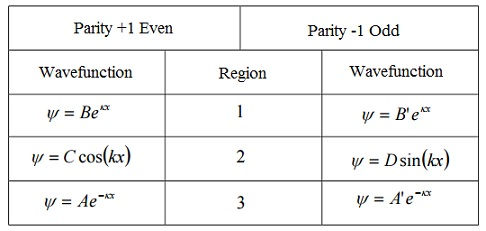Tutorsglobe: A way to secure high grade in your curriculum (Online Tutoring)